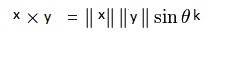# Vector product of two vectors

the vector product is a binary operation on two vector in three-dimensional space and is denoted by the symbol ×. Given two linearly independent vectors x and y, the cross product, x × y, is a vector that is perpendicular to both and therefore normal to the plane containing them. If two vectors have the same direction (or have the exact opposite direction from one another, i.e. are not linearly independent) or if either one has zero length, then their cross product is zero. More generally, the magnitude of the product equals the area of a parallelogram with the vectors for sides; in particular, the magnitude of the product of two perpendicular vectors is the product of their lengths. The cross product is anticommutative (i.e. x × y = −y × x) and is distributive over addition (i.e. x × (y + z) = x × y + x × z). The space together with the cross product is an algebra, which is neither commutative nor associative. The cross product of two vectors x and y is defined only in three-dimensional space and is denoted by x × y.